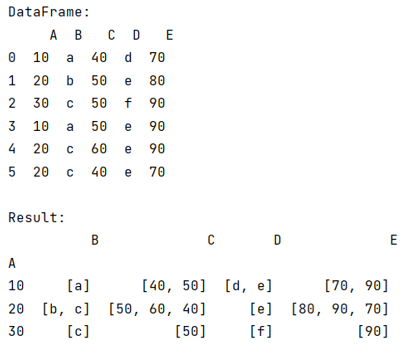# Python - Can pandas groupby aggregate into a list, rather than sum, mean, etc?

Given a Pandas DataFrame, learn that can we groupby aggregate into a list rather than sum?
Submitted by Pranit Sharma, on August 03, 2022

Pandas is a special tool that allows us to perform complex manipulations of data effectively and efficiently. Inside pandas, we mostly deal with a dataset in the form of DataFrame. DataFrames are 2-dimensional data structures in pandas. DataFrames consist of rows, columns, and data.

Here, we are going to learn that can we groupby aggregate into a list rather than a sum. We will try to understand this by applying the aggregate function inside groupby method.

## pandas.DataFrame.groupby() Method

On the other hand, pandas.DataFrame.groupby() is a simple but very useful concept in pandas. By using groupby, we can create a grouping of certain values and perform some operations on those values.

The pandas.DataFrame.groupby() method splits the object, apply some operations, and then combines them to create a group hence a large amount of data and computations can be performed on these groups.

We will first create a DataFrame then we will use groupby inside which we will use the lambda function to convert this result into a list.

Let us understand with the help of an example,

## Python code to demonstrate 'can pandas groupby aggregate into a list, rather than sum, mean, etc'

```# Importing pandas package
import pandas as pd

# Creating a dictionary
d = {
'A':[10,20,30,10,20,20],
'B':['a','b','c','a','c','c'],
'C':[40,50,50,50,60,40],
'D':['d','e','f','e','e','e'],
'E':[70,80,90,90,90,70]
}

# Creating a dataframe
df = pd.DataFrame(d)

# Display Dataframe
print("DataFrame:\n",df,"\n")

# Using groupby
df2 = df.groupby('A').aggregate(lambda x: x.unique().tolist())

# Display result
print("Result:\n",df2)
```

Output:Languages: » C » C++ » C++ STL » Java » Data Structure » C#.Net » Android » Kotlin » SQL
Web Technologies: » PHP » Python » JavaScript » CSS » Ajax » Node.js » Web programming/HTML
Solved programs: » C » C++ » DS » Java » C#
Aptitude que. & ans.: » C » C++ » Java » DBMS
Interview que. & ans.: » C » Embedded C » Java » SEO » HR
CS Subjects: » CS Basics » O.S. » Networks » DBMS » Embedded Systems » Cloud Computing
» Machine learning » CS Organizations » Linux » DOS
More: » Articles » Puzzles » News/Updates